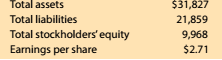Chapter 8, Problem 8.9.2MBA

Chapter
Section
Textbook Problem

Debt and price-earnings ratios Lowe's Companies Inc. (LOW) operates over 1,800 home improvement retail stores and is a competitor of The Home Depot (HD). The following data (in millions) were adapted from a recent financial statement of Lowe's:2. Given your answer lo pan (1), what is the ratio of Stockholders' equity to total assets? Round to one decimal place.

To determine

Concept Introduction:

Debt Ratio:

Debt ratio is the relationship between the Total liabilities and Total Assets of a corporation. Debt ratio shows the part of assets financed by debts. It is calculated by dividing total liabilities by total assets. The formula of debt ratio is as follows:

Debt Ratio = Total LiabilitiesTotal Assets

To Calculate:

The Stockholder's Equity to total asset ratio using the Debt Ratio

Explanation

The Stockholder's Equity to total asset ratio using the Debt Ratio is calculated as follows:

 $in Millions Total Liabilities (A)$21,859

Still sussing out bartleby?

Check out a sample textbook solution.

See a sample solution

The Solution to Your Study Problems

Bartleby provides explanations to thousands of textbook problems written by our experts, many with advanced degrees!

Get Started

THE ACCOUNTING EQUATION Using the accounting equation, compute the missing elements.

College Accounting, Chapters 1-27 (New in Accounting from Heintz and Parry)

MIRR Refer to problem 11-1. What is the projects MIRR?

Fundamentals of Financial Management, Concise Edition (MindTap Course List)

WACC Midwest Electric Company (MKC) uses only debt and common equity. It can borrow unlimited amounts at an int...

Fundamentals of Financial Management, Concise Edition (with Thomson ONE - Business School Edition, 1 term (6 months) Printed Access Card) (MindTap Course List)